### Fisher’s z Transformation

Subsections:

For a sample correlationthat uses a sample from a bivariate normal distribution with correlation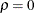, the statistic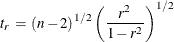has a Student’sdistribution with (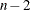) degrees of freedom.

With the monotone transformation of the correlation(Fisher, 1921)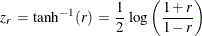the statistichas an approximate normal distribution with mean and variance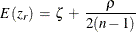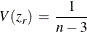where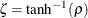.

For the transformed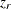, the approximate variance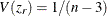is independent of the correlation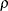. Furthermore, even the distribution ofis not strictly normal, it tends to normality rapidly as the sample size increases for any values of(Fisher, 1973, pp. 200–201).

For the null hypothesis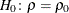, the
-values are computed by treating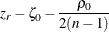as a normal random variable with mean zero and variance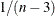, where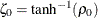(Fisher 1973, p. 207; Anderson 1984, p. 123).

Note that the bias adjustment,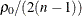, is always used when computing-values under the null hypothesisin the CORR procedure.

The ALPHA= option in the FISHER option specifies the value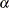for the confidence level, the RHO0= option specifies the value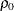in the hypothesis, and the BIASADJ= option specifies whether the bias adjustment is to be used for the confidence limits.

The TYPE= option specifies the type of confidence limits. The TYPE=TWOSIDED option requests two-sided confidence limits and a-value under the hypothesis. For a one-sided confidence limit, the TYPE=LOWER option requests a lower confidence limit and a-value under the hypothesis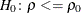, and the TYPE=UPPER option requests an upper confidence limit and a-value under the hypothesis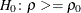.

#### Confidence Limits for the Correlation

The confidence limits for the correlationare derived through the confidence limits for the parameter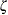, with or without the bias adjustment.

Without a bias adjustment, confidence limits forare computed by treating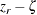as having a normal distribution with mean zero and variance.

That is, the two-sided confidence limits forare computed as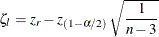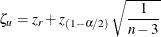where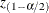is the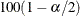percentage point of the standard normal distribution.

With a bias adjustment, confidence limits forare computed by treating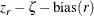as having a normal distribution with mean zero and variance, where the bias adjustment function (Keeping, 1962, p. 308) is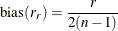That is, the two-sided confidence limits forare computed as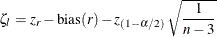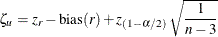These computed confidence limits of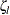and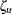are then transformed back to derive the confidence limits for the correlation: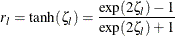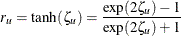Note that with a bias adjustment, the CORR procedure also displays the following correlation estimate: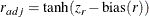#### Applications of Fisher’s z Transformation

Fisher (1973, p. 199) describes the following practical applications of thetransformation:

• testing whether a population correlation is equal to a given value

• testing for equality of two population correlations

• combining correlation estimates from different samples

To test if a population correlation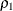from a sample of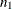observations with sample correlation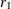is equal to a given, first apply thetransformation toand: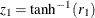and.

The-value is then computed by treating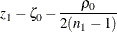as a normal random variable with mean zero and variance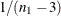.

Assume that sample correlationsand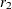are computed from two independent samples ofand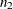observations, respectively. To test whether the two corresponding population correlations,and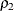, are equal, first apply thetransformation to the two sample correlations:and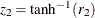.

The-value is derived under the null hypothesis of equal correlation. That is, the difference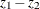is distributed as a normal random variable with mean zero and variance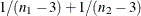.

Assuming further that the two samples are from populations with identical correlation, a combined correlation estimate can be computed. The weighted average of the correspondingvalues is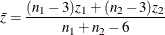where the weights are inversely proportional to their variances.

Thus, a combined correlation estimate is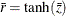and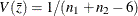. See Example 2.4 for further illustrations of these applications.

Note that this approach can be extended to include more than two samples.